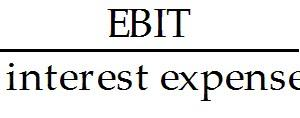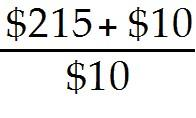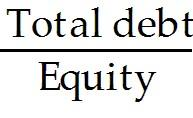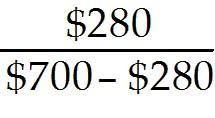# Essay. Write your answer in the space provided or on a separate sheet of paper

 Page 1/6 Date 03.08.2017 Size 414.29 Kb.

ESSAY. Write your answer in the space provided or on a separate sheet of paper.

1)

In 2010, a biotechnology firm, DNA Map Inc., had \$700 million of assets and \$280 million of liabilities. Earnings before interest and taxes were \$215 million, interest expense was \$10 million, and the tax rate was 32%.
(a) Calculate the times-interest-earned.

(b) Calculate the debt-to-equity ratio.

(c) Calculate the net margin.

2)

The following data are available for two companies, Apple and Oracle, all stated in thousands of dollars.

 Description Apple Oracle Total revenue \$42,905,000 \$23,253,000 Earnings before interest and taxes 12,066,000 8,464,000 Interest expenses 0 630,000 Earnings before tax 12,066,000 7,834,000 Taxes at 40% 3,831,000 2,241,000 Earnings after tax (Net income) 8,235,000 5,593,000 Debt \$15,861,000 \$22,326,000 Equity 31,640,000 \$25,090,000

(a) Calculate each company's return on equity (ROE) and return on asset (ROA)

(b) Which company has performed better in terms of profitability?

(c) If two companies were combined (merged), what would be the impact on the results on ROE? Under what conditions would such a combination make sense?

3)

The following shows selected comparative statement data for Dell Corporation. All financial data are as of January 31 in millions.

 2009 2008 Total revenue \$61,101 \$61,133 Cost of revenue \$50,144 \$49,462 Net income \$2,478 \$2,947 Account receivables \$4,731 \$7,693 Inventory \$867 \$1,180 Current assets \$20,151 \$19,880 Long-term liabilities \$7,370 \$5,206 Total assets \$26,500 \$27,561 Total common shareholders' equity \$4,271 \$3,735

For year 2009,

(a) What is the profit margin?

(b) What is the total asset turnover?

(c) What is the current ratio?

(d) What is the return on asset?

(e) What is the return on common shareholders' equity?

4)

The following data were taken from the income statements of Broadcom Corporation (BRCM).

 2009 2008 Total revenue \$4,658,125 \$3,776,395 Beginning inventory \$231,313 \$202,794 Purchases \$510,711 \$566,145 Ending inventory \$366,106 \$231,313

Compute for each year the inventory turnover ratio and what conclusions concerning the management of the inventory can be drawn from the data?

5)

Given the following facts, complete the balance sheet
Given

• Collection period 45.6 days

• Current ratio 1.38 times

• Quick ratio 1.13

• Inventory turnover ratio 23.5 times

• Time-interest-earned ratio 5.00

• Asset Turnover ratio 1.63

• Return on common equity 28.2%

• Gross margin 32.5%

• Net Margin 7.50%

• Cash \$300

• Total sales revenue \$4,000

• Total current assets \$1,100

∙ Total assets \$2,450

Find

∙ Shareholders’ equity

∙ Current liabilities

∙ Account receivables

∙ Net income

1)

(a) Time-interest-earned ratio === 22.5 times
(b) Debt to equity ratio === 66.67%